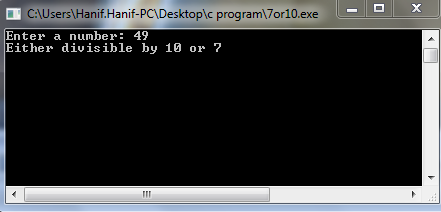# C Program to Check the Number is Divisible by 10 and 7 or Not

## In this example, you will learn the number is divisible by 10 or 7 or not (entered by the user).Divisible by 10 or 7 or Not

### This program uses if - else statement to check the number is divisible by 10 or 7 or not.

#include<stdio.h>
main()
{
int x;
printf("Enter a number");
scanf("%d",&x);
if(x%10==0 && x%7==0)
{
printf("divisible by 10 and 7");
}
else if(x%10==0 ||  x%7==0)
{
printf("Either divisible by 10 or 7");
}
else
{
printf("neither divisible by 10 or 7");
}
getch();
}Divisible by 10 or 7
The entry number is 49, and the output will show either divisible by 10 or 7. It means the entry number divisible either 10 or 7, not both.

The entry number is 55, and the output will show neither divisible by 10 or 7. It means the entry number is not divisible by 10 or 7.

The entry number is 70, and the output will show divisible by 10 and 7. It means the entry number is divisible by both number 10 and 7.